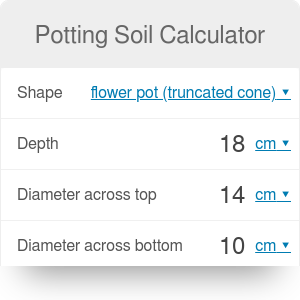Shape
rectangular
Length
in
Width
in
Depth
in
Quantity
US qt

# Potting Soil Calculator

By Hanna Pamuła, PhD candidate

Potting soil calculator allows you to measure the volume of soil needed for your container. Whether you want to have a raised bed in your garden or rearrange your blooming balcony, use the calculator to estimate how much soil you need to prepare. Choose between three types of containers, calculate your needs and go gardening!

## Container shape

There are many different containers types on the market. In our potting soil calculator, you can select between three different shapes. It finds the volume according to the formulas:

• rectangular - e.g. raised bed soil containers

volume of rectangular cuboid: `volume = depth * length * width`

• round

volume of a cylinder: `volume = π * R² * depth` , where `R` is a radius

• flower pot in the shape of truncated cone

volume of a truncated cone: `volume = (1/3) * π * depth * (r² + r * R + R²)`, where `r` is a radius of the base of flower pot, and `R` of top surface radius

If you want to estimate the weight or cost of topsoil, use our soil calculator.

## How much soil do I need?

Let's imagine that you want to grow herbs. You have couple small flower pots, and you don't know how much soil do you need. Potting soil calculator can help in that problem:

1. Choose the shape of the container. In our case, it's a flower pot, so we need to calculate the volume of truncated cone.
2. Enter the dimensions. For example, assume we have pots with the diameter across top equal to 4 inches, bottom base diameter of 3.2" and height of 2.75". Don't forget that you can select different units, such as centimeter, feet etc.
3. Set the quantity of the flower pots. We want to plant oregano, basil, and thyme, so we need to put three in the quantity field.
4. The volume of soil required is equal to displayed value.

Our calculator is a flexible tool, so you can calculate everything the other way round: if you have a bag of soil and size of your containers, you can estimate how many pots can be filled.

Remember that obtained result represents the volume for the full container. Sometimes you want to fill the container to 4/5, or you already have a plant or tree with the roots and then you will need less soil. The amount of soil may also change as you squeeze it or leave it looser, so presented calculations are only a good estimator.

Hanna Pamuła, PhD candidate

## Get the widget!

Potting Soil Calculator can be embedded on your website to enrich the content you wrote and make it easier for your visitors to understand your message.

It is free, awesome and will keep people coming back!